# Analysis of Radar and Air-Visual UFO Observations on 24 October 1968 at Minot AFB, North Dakota, USA

Claude Poher, Ph.D.

## Part 5. The Ionized Cloud Surrounding the UFO

### 5.1. The Air-Visual UFO Observation from the Perspective of the B-52

According to the descriptions of the pilots, the B-52 executed a 90-degree left turn onto the base leg at the point where it was closest to the UFO on or near the ground. At this time the B-52 was at 3200 feet altitude (MSL), or about 450 meters (1500 feet) above the ground, and its speed was 180 knots (92.6 m/s). We can assume that pilot Major Partin, seated on the left side of the cockpit, centered the turn over the UFO in order to better observe it. This would place the UFO at the focal point to the axis of the wing and in front of the left lateral windows of the cockpit.Figure 54. Two views from copilot Capt. Runyon’s perspective looking across the B-52 cockpit. Runyon viewed the UFO on or near the ground through the pilot’s windows during the 90o left turn to the base leg.

A turn made at the standard rate of 180 degrees per minute corresponds to a turn radius of 20 seconds of flight, which is 1852 meters (1 nm). We can multiply 20 seconds by π (3.14) to arrive at 63 seconds for a 180-degree turn. Therefore, the duration of a 90-degree turn performed at this standard rate is 30 seconds. However, copilot Runyon reported having observed the UFO across the cockpit through the pilot’s windows for only about 10 seconds. This suggests that Partin performed the turn much closer to the UFO and with a larger incline than would be normal for a standard turn.

Let us call R the turn radius. The distance traveled by the B-52 in the course of the 90 degree turn is simply π R / 2, and the time (T) needed to go through this distance is T = π R / (2 . 92.6) = 10 seconds. We can easily deduce that R = 590 meters. The distance D separating the pilots from the UFO in these turn conditions is resolved by:

• D 2 = (450) 2 + (590) 2 , which gives: D = 740 meters (2428 feet).Figure 55. Hypothetical 30-degree bank angle during the left turn onto the base leg.

However, such a steep turn is not possible for a B-52 at low speed (180 kts). We must concede that both pilots could not have observed the UFO throughout the entire turn (30 seconds), but only for about one third of the total duration (10 seconds) with a necessary turn radius of about one nm. This observational turn was centered in order to pass as close as possible over the top of the UFO. The trajectory is quite compatible with the recollections of the witnesses, concerning its duration and timing. For example, Runyon recalls:

“I would probably put ten seconds in the range because we flew down the side of it for about two or three, maybe four seconds… . Major Partin started his turn just as we got abreast of the end of it and turned almost over the top of the thing. I'm sure we were told to turn by the [radar] ground controllers so they knew exactly where we were in relation to it.” 

### 5.2. Dimensions of the UFO According to B-52 Pilot Partin

In accordance with our previous calculations, the incline of the airplane would have been:

• Incline = Arc Tg (450 / 590) = 37 degrees.

This is a significant angle of incline for a B-52 at low speed during approach. At such an angle, the weight of the plane multiplied by the load factor (caused by the centrifuge force) must be compensated with a corresponding lift increase during the turn. In this instance, the load factor would have reached 1.26 g, making it necessary to augment the lift by 26% in comparison with horizontal flight. As a result, even if the incline of the B-52 was less, we can assume that Partin paid close attention to the controls of the aircraft, which would only allow stealthy looks towards the UFO. However, in his official report, he made two drawings of what he saw. His first drawing is a circle representing an orange ball of light with an oval ring of soft white light extending to the right side in which the ratio of the axes is 3.7.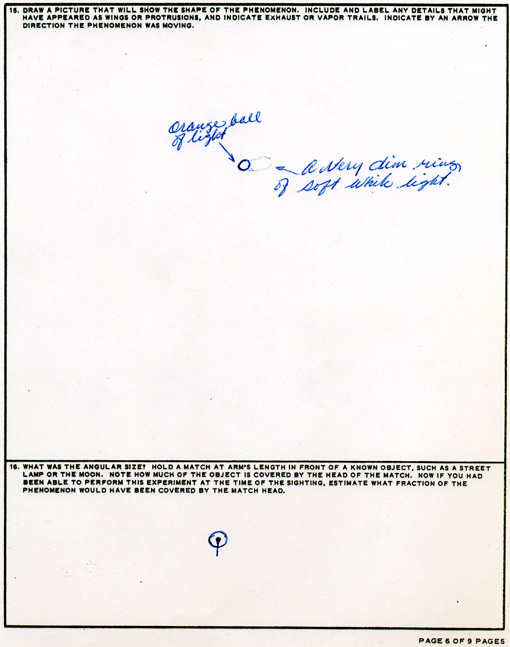Figure 56. Two drawings by B-52 pilot James Partin from his AF-117 dated 30 Oct. 1968. In a 2001 interview, he recounted that at 1500-1700 feet altitude a house on the ground appeared to be about the size of a die, or a Monopoly house, and the UFO appeared to be much larger than that.

His second drawing features the angular diameter of the orange ball of light by estimating what fraction of the visible phenomenon a match head would have covered at arm’s length. The drawing indicates that its diameter, as seen from the B-52, was 4 times the width of the end of match (4 mm) held at arm’s length (60 cm). We can deduce that the angular diameter, expressed in radians, was 1.6 / 60. When we multiply this by the probable distance of calculated observation at 740 meters (2428 feet), we get the following dimensions of the orange ball of light:

Diameter of the UFO = 1.6. 740 / 60 = 20 meters (65 feet)

Length of the UFO = 20 x 3.7 = 73 meters (240 feet)

### 5.3. Dimensions of the UFO According to B-52 Copilot Runyon

During the downwind leg of the traffic pattern, copilot Captain Bradford Runyon completed the various landing checklists, and not being solely responsible for piloting the aircraft, would have been in the better position to view the UFO throughout the 90-degree turn. Here is his drawing: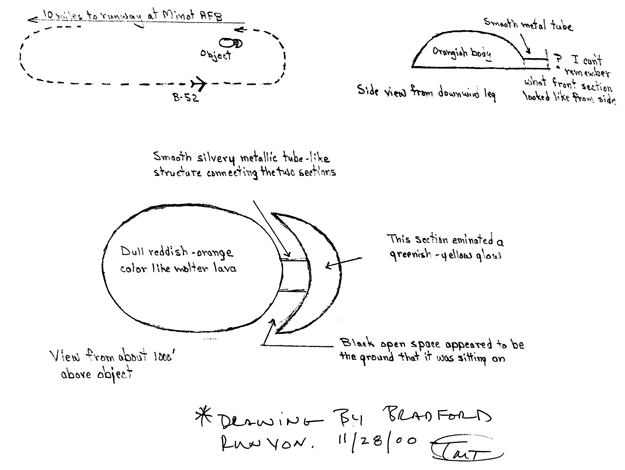Figure 57. Drawings of the UFO by copilot Bradford Runyon made in November 2000. Project Blue Book investigators did not interview Runyon in the course of the official investigation.

In Runyon’s estimation, the UFO measured about 200 feet (60 meters) in length, 100 feet (30 m) in width, and 50 feet (15 m) in height. The dimensions estimated by the two pilots are relatively close, considering the brevity of their observation (10 seconds). Both estimated the dimensions by comparing them with houses and farm buildings. For example, at first Runyon thought he was seeing the side of a barn; whereas Partin affirmed that the UFO was much larger than a house

In addition, Partin provided angular dimensions, which allow us to calculate the real dimensions according to the reconstruction of the trajectory of the B-52. It is unlikely that the similar dimensions are chance, since these are seasoned professionals specifically trained in the recognition of targets during a penetration at low altitudes under any conditions. In addition, the visibility close to the ground was unobstructed for 25 miles. Thus, both pilots were able to clearly view the object.

### 5.4. Dimensions of the UFO According to the Radar Echo

The radar onboard the B-52 provides its own unique description of the UFO and its movements, which are preserved for analysis by the consecutive radarscope photographs. In this case, these photographs are first-generation prints from the original negative film of the B-52 radar camera, a copy of which were retained by the 5th BMW intelligence officer SSgt. Clark at Minot AFB. We can enlarge the image since it was scanned with a resolution of 300 pixels per inch (dpi) from the original 8 X 10 photographic print.

Here is the central region referred to as the “TR (transmit/receive) hole,” or “altitude hole” of photo 773:Figure 58. The radar echo of the UFO in radarscope photo 773 (negative image).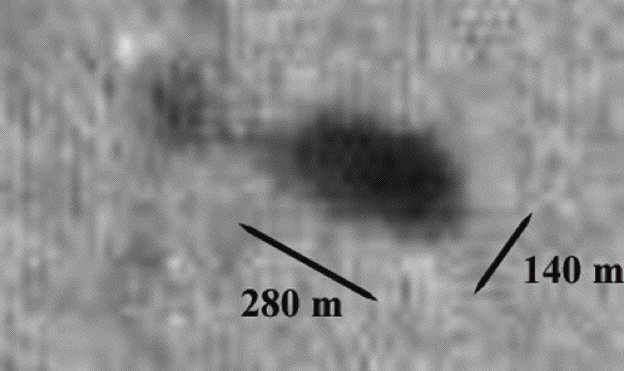Figure 59. Enlargement of the UFO echo. (280 m = 920 feet; 140 m = 460 feet).

Since the B-52 radar allows us to establish distance with accuracy in the order of 30 meters, we have an idea of the considerable size of the echo of the UFO. The main body of the echo has a length in the order of 250 / 280 meters (820 / 918 feet) and a width in the order of 140 meters (459 feet). Towards the left of the main body of the UFO there appears a barely resolved bridge more than 150 meters (492 feet) long, and a second, poorly resolved body in the order of 100 meters (328 feet) diameter. A specific software program allows us to illustrate the outline of the echo by defining the perimeter of the object at the threshold of the atmospheric background.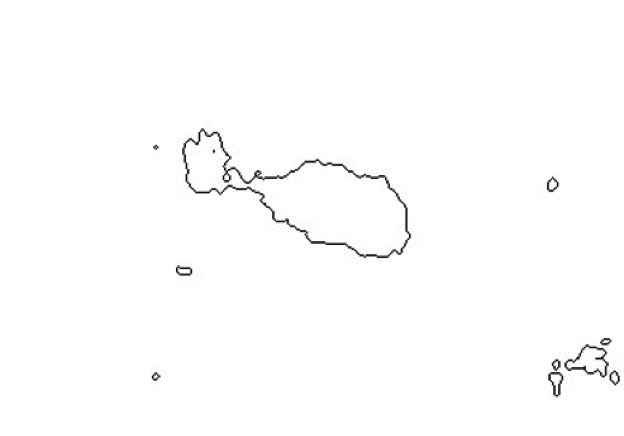Figure 60. Outlines of the threshold of the UFO echo in photo 773.

In the outline, we see the general shape of the echo captured by the radar. This shape is similar to the drawings of the object by Captain Runyon at a distance of approximately 740 meters (2428 feet). However, both pilots estimated the dimensions of the object to be about a quarter of those that are measured by this radar echo. Let us examine this discrepancy in more detail. A more detailed analysis, which outlines the photographic darkening with various thresholds of intensity in false color, reveals a variable density of the echo from its edges to its center.Figure 61. Outlines of various thresholds of the UFO echo in photo 773.

We can conclude from the previous figure that the echo does not appear as though the return of the radar waves were the result of a metallic surface having the equivalent dimensions as the echo. It seems that the reflection coefficient (i.e., the radar albedo) increased as more microwaves entered inside the volume of the echo. What the radar “paints” on the scope is actually the intensity of the microwave signal returned by the target, rather than the actual target itself. Therefore, we must examine the properties of electromagnetic wave propagation while being as concise as possible.

### 5.5. Using Theoretical Physics to Explain the Luminous Halo Phenomenon

In many cases, when UFOs are observed in nocturnal conditions witnesses report that they are surrounded by an intense luminosity, often as a function of extreme acceleration. Over the course of 30 years of theoretical physics studies, I have been able to demonstrate that the phenomenon is actually a secondary atmospheric effect caused by the propulsion field of the UFO.

My Universons’ theory hypothesizes that the effect is a result of a quantified gravitational field that interacts with elementary particles of matter, called Universons. These Universons consist of a natural energetic flux containing kinetic momentum. This theory explains inertia and gravitation, provided that Universons have a particular behavior (an assumption that will not be explored in this present study).

Universons’ theory also postulates the existence of a constant cosmological acceleration, which would be added to any accelerated matter as a result of the expansion of the Universe. In fact, this cosmological acceleration has apparently been observed by NASA-JPL in the trajectory of the interplanetary space probes Pioneer 10 and 11. The same cosmological acceleration also explains remarkably well an astronomical mystery observed by Edwin Hubble in 1950, specifically the constant orbital speed of stars, a speed that occurs independent of the orbital radius in galaxies. Therefore, we have several observational confirmations of the scientific predictions of Universons’ theory.

The application of the natural flux of Universons could theoretically be applied to numerous applications, particularly to propelling space vehicles. For example, if it were possible to control the trajectory of Universons re-emitted naturally by the matter of the vehicle, we could create inertia in an entirely unique way. Further, theoretical studies have revealed how such a propulsion method would permit the same acceleration for all elementary particles of the matter associated with the spacecraft, including the occupants, thereby eliminating the disadvantages of inertial effects and particularly the mechanical constraints linked to acceleration. In other words, these studies show that this type of propulsion would be able to produce considerable acceleration of the vehicle (several hundred g’s) without vital risk to the passengers.

Given these conditions, the vehicle could attain a relativist speed within a short time period. In effect, at 600 g acceleration the speed would be 93 % of the speed of light in 13 hours. If the speed of the vehicle becomes relativistic, we know that the onboard clock would decelerate considerably in comparison with the clock located on the planet of departure. In this case, the lifespan of the occupants of the vehicle would not be a limit to the surmountable distances, since the vehicle could travel between all points in the Galaxy during the lifetime of the occupants.

The only theoretical limitation in an interstellar voyage using this mode of propulsion would concern energy. However, the energy onboard the vehicle could theoretically be extracted from the natural field of the Universons. There are numerous natural examples where such extraction of energy is accomplished, including the kinetic energy of the solar system orbiting around the Galactic center. [Our kinetic energy is 30 billion joules per kg of matter, including the matter with which our human bodies are composed]. Therefore, these theoretical considerations are reinforced by the results of astronomical observatories and space trajectography data stations, and present a novel scientific status in the practicability of an interstellar voyage by intelligent beings.

In addition, these theoretical studies reveal very particular characteristics of vehicles with this type of propulsion. Let us assume that these characteristics are precisely those described by witnesses who have observed UFOs for decades. The two primary theoretical characteristics of these observations are the ability for stationary flight, or in very powerful acceleration with an absence of a sonic boom in spite of a supersonic speed in the dense atmosphere, and the presence of a bright atmospheric halo that encompasses the vehicle.

### 5.6. The Luminous Halo Encircling the UFO

With regard to the unidirectional flux of Universons, a propelling flux that is able to push equally on all elementary particles of the UFO would result in all of the electrons of the atoms being subjected to unidirectional acceleration as a result of the field, which is vectorially added to the acceleration caused by the electric attraction of the nucleus. This effect is not limited to the vehicle’s atomic structure, but also includes the atmospheric air encircling it. That is, the atoms of atmospheric gas are also subjected to the significant pressure of this propulsive flux. Given these conditions, we would likely observe a significant distortion of the electronic orbits in the atoms constituting the gases of the atmosphere. This orbit would adopt an oblong shape under the influence of the external propelling field not unlike a rugby ball:Figure 62. In a constant external propelling field, the electronic orbits of atoms are distorted, creating a negative space charge where the orbits are most distant from the nucleus.

Naturally, the more distant the atoms of gases are from the UFO, the less intense the phenomenon. However, close to the UFO the propelling flux would be so intense that it can tear off one or more electrons from the atom, especially during strong acceleration. The propelling flux partially ionizes the air encompassing the UFO. The free electrons collide with other atoms in the pressure that exists at ground level, where the densities of atoms are 2.65. 10 25 per cubic meter. Of course, the free electrons are particularly attracted to the positive ions that have lost one or more electrons earlier. Therefore, we have a double phenomenon. On the one hand, we witness an extraction of electrons in the gas due to the propelling field, and on the other hand, we observe a recombination of electrons with ions.

The recombination of electrons is accompanied by an emission of photons of light, which is essentially the way light is produced in nature. Another term for this process is radiative recombination in which the emitted photons of light have a characteristic color depending on the type of ionized atoms in the atmosphere (i.e., nitrogen, oxygen, carbon dioxide, xenon) and the kinetic energy of the liberated electrons. Curiously, the colors of the radiative recombination photons of the atoms constituting the atmosphere of the Earth are in our case exactly what the ground witnesses described. However, our concern is not the light issued by the atoms of partly ionized air encircling the UFO, but rather, the behavior of radar waves in this partly ionized gas.

### 5.7. The Behavior of Electromagnetic Waves in Ionized Gas

When electromagnetic waves began to be used for radio communications over large distances, a fluctuating phenomenon referred to as fading was observed. Fading is caused by a reflection of the waves on an agitated layer of gas in the ionosphere. As a result of ionospheric reflection, it was possible to communicate by radio with antipodes in a series of successive rebounds of the wave between the conductive ionosphere and the ground of the Earth. In the beginning, radio waves were very long, prior to the advent of electronic tubes, which allowed the generation of high frequency waves and amplification of the reception.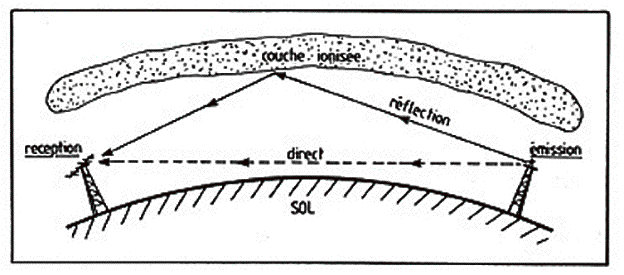Figure 63. The electromagnetic waves reach the reception antenna by two separate interfering paths. One by direct propagation in free space, and a second reflected on the agitated ionosphere.

The ionosphere acts as a mirror only for electromagnetic waves lower than a critical frequency. When the emitted frequency F1 is larger than critical frequency Fc, the wave is simply transmitted through the ionosphere and nothing is returned. When emitted frequency F2 is smaller than Fc, the wave is reflected completely and returned to the receiver. This effect is used every day by short wave band communications; in the study and prediction of ionospheric conditions; and scientific research to understand the plasma physics of the solar-terrestrial interaction of the Earth’s atmosphere and magnetic field with the solar wind.Figure 64. Principle of an ionospheric sounder constituted by a transmitter and a receiver placed very close, sending and receiving pulsed radio waves in the vertical direction

Fc changes according to local time and from one day to the next, due to natural conditions. In effect, it is such that:

(2 π Fc)2 = N e2 / Σo me         (48)

In this expression, e is the charge of the electron, me its mass, Σo is the dielectric constant of vacuum, and N is the average number of free electrons per cubic meter in the ionosphere. Basically, we use the following equation:

Fc2 = 80.7 N         (49)

As a result:

Nc = 0.0124 Fc2         (50)

Relation (50) allows us to determine the average electronic density (free electrons per cubic meter) of the ionosphere by measuring the critical frequency. The ionosphere is a vacuous region of the upper atmosphere from 60 to about 1000 km in altitude. At this altitude the ultraviolet photons of light from the sun are not entirely absorbed by the molecules of gas, as is the case in low layers of the atmosphere. In fact, these photon rays are able to tear off electrons from the atoms of gas. However, the density of gas is weak and the free mean path of an electron is very large. Thus, the amount of electrons that are recaptured by the ions is significantly reduced in high altitudes compared to low altitudes. The gas is therefore a mixture of positive ions and free electrons of an electronic density N.

Of course, at night, there are no solar ultraviolet photons. Therefore, electronic density drops quickly at sunset. Further, according to seasonal changes, the ultraviolet rays are more or less tipped-up in comparison with the zenith, affecting the degree of ionization. Finally, the amount of ultraviolet rays reaching earth is variable according to changing solar activity. The electronic density N, and consequently Fc are therefore varying phenomena. Fc can in fact vary by a factor of 4 between day and night in winter, and by a factor of 2 between day and night in summer. It also varies altitudinally throughout the successive layers of the ionosphere. Generally, the critical frequency of the ionosphere varies from 3 to 15 MHz according to location and time of day. Therefore, the ionized gas, sometimes called plasma, has specific properties that deviate electromagnetic waves. Its index of refraction is larger when the specific density of the free electrons N is also larger. Consequently, the path followed by the electromagnetic waves is curved, because the electronic density varies vertically.Figure 65. By crossing successive layers of variable refraction index the EM waves are deviated following a curved path.

For waves having less than the critical frequency, if the curvature angle of the path attains 180 degrees the wave returns to its starting point. Therefore, the critical frequency is the one for which the electromagnetic wave is completely reflected by the ionosphere. Space research and exploration that operates above the ionosphere utilizes communication and telemetry frequencies greater than the critical frequency, which is one of the reasons why space telecommunications use frequencies of several gigahertz. However, even when we use frequencies superior to the critical frequency of the ionosphere to communicate with artificial satellites in orbit there are always deviations of the waves caused by the ionosphere.Figure 66. When we want to communicate with an artificial satellite by means of a very directive beam of radio waves we need to take into account the ionospheric refraction, which deflects the electromagnetic waves path.

These considerations of ionospheric deviations of the electromagnetic waves are quite significant as we are about to see.

### 5.8. The Effect of Air Ionization on Radar Waves

The electronic density of the ionosphere would be significantly less than the atmosphere encompassing a UFO in flight. In this instance, the ionization of gas is not caused by the interaction of ultraviolet photons, but rather by the pressure of the flux of Universons that augment the lift and acceleration of the UFO. Consequently, the ionization is so intense that the luminosity emitted by the atmosphere is considerable, particularly during a powerful acceleration of the UFO. Theoretical physics allows us to calculate the intensity of the light emitted according to the propelling flux. In this instance, the ground observers at Minot AFB are unanimous in describing a very large UFO, which alternated color from a very bright white to orangish-red and green. It would seem the rate of ionization of air encompassing the UFO was considerable.

Certainly, we do not know how to reproduce such levels of ionization of air at an atmospheric pressure of 1 bar. However, we can extrapolate experimental and theoretical knowledge at lower pressures in the ionosphere. We know that the B-52 radar transmitted pulses at a frequency of about 9000 MHz. We can therefore extrapolate the complete reflection of these radar waves and their corresponding electronic density. In order to accomplish this task, let us apply Fc = 9000 MHz to equation (50):

Nc = 0.0124 Fc2 = 0.0124 (9.109)2         (50 bis)

We get:

• Nc = 1018 elections per cubic meter.

At the time of the radarscope photos, the B-52 was at an altitude of 3000 meters MSL. On average, we know that at this altitude the atmosphere contains 1.9 . 10 25 molecules or atoms per cubic meter. As a result, the calculated ionization (compare this content with Nc) is only partial and concerns one ionized atom per 19 million. However, this feeble ionization rate is theoretically sufficient to completely reflect a radar wave of 9000 MHz and to return an echo as intense as the ground echo beneath the B-52. Here then is the probable schema of what took place at Minot on 24 October 1968:Figure 67. A zone of highly ionized air, which emits a powerful diffuse light encircling the UFO. The electronic density in the UFO halo is such that in the partly ionized gas the radar waves are strongly deviated, even completely reflected. (AIR IONISE = Ionized air. ONDES DEVIEES = Deviated waves).

The schema of the previous figure demonstrates that lateral radar waves, which should normally not attain the reflective surface of the UFO, are in fact highly deviated and return to the radar in such a way that they augment the apparent size of the radar echo compared to the witness estimates. The radarscope photographs are an interesting confirmation of our hypothesis, since they allow us to determine the order of magnitude of the minimal electronic density that existed in the air encompassing the UFO (about a billion-billion electrons per cubic meter), as well as the proportion of atoms ionized (about one atom ionized for 19 million atoms remaining neutral).

### 5.9. The Loss of VHF Transmission

The dimensions of the radar echo (280 x 140 meters) are not the actual dimensions of the UFO. The radar dimensions represent a volume of air in which the electronic density was sufficient to deviate the 9000 MHz radar waves. However, the presence of free electrons did not limit itself to the region surrounding the UFO, which is approximately 15 % of the distance separating the B-52 and the UFO during the sequence of radar photographs.

It is probable that the electronic density diminished progressively at some distance from the UFO in a way proportional to the square or cube of distance. Since the B-52 was situated about seven times farther away from the UFO than the zone of ionization, it is probable that the electronic density near the B-52 was equal to at least 1/50 or 1/350 of the electronic density of the cloud of ion s that produced the radar echo. Evidently there was a considerable density of electrons around the B-52, probably in the order of at least 1015 electrons per cubic meter, (which is the previous value of Nc divided by 350). Consequently, the VHF communications antenna of the B-52 operating at 270 MHz was immersed in a relatively conducting gas. This did not hinder reception of the VHF communications from RAPCON, because the corresponding electrical field was insufficient to perturb the atmospheric ions at only a few millivolt VHF voltage at the receiving antenna.

However, when the B-52 copilot pressed the button on his microphone, the transmitter onboard the B-52 was sending its entire power to the quarter wave antenna. In this instance, the amplitude of the antenna’s local electrical field was increased, which immediately attracted the ions and the electrons encircling the antenna. With a thick layer of ions encircling the transmission antenna, all of the transmitting power was absorbed in the agitation of ions and the heating of air. Therefore, the radiated power was very weak. In other words, the radiating impedance of the antenna was bypassed by the bath of ions as if it had been plunged into a conducting fluid driver such as water. Another way to understand this phenomenon is to consider the critical frequency of ionized air by applying N = Nc /350 to equation (49):

Fc2 = 80.7 N = 80.7 . 2.9 . 1015 ==> Fc = 484 MHz         (49 bis)

In this case the critical frequency (484 MHz) is superior to the frequency of communications (270 MHz) and the wave is returned and absorbed rather than being transmitted. In addition, the transponder transmitted without a problem because it operated above the critical frequency over 1 GHz. It is remarkable that these considerations of plasma physics are completely consistent with many described facts in this case.

### 5.10. Discussion 2: Photometric Study and the Ionized Cloud Surrounding the UFO

Thanks to the fact that Tom Tulien sent full-sized photographic copies of the original first-generation 8 X 10 prints, we have been able to study the variation of the photo darkening (Var) along the right side of the radar ground echo in photo 783. These variations allowed us to confirm the following equation for the radar screen brightness Sb of the echoes:

Sb = KVar = K sin2 (φ) sin2 (α) / D4         (51)

For the different ranges D indicated in Figure 51 we have the following results:

• For D = 2.5 nm range, Var = 0.2768.
• For D = 3 nm range, Var = 0.8976.
• For D = 4 nm range, Var = 3.1395.
• For D = 5 nm range, Var = 6.5332.

We can normalize these values for the 2.5 nm range and call them Var1, which means that we have increased all the previous values by a factor 3.613:

• For 2.5 nm range, Var1 = 1.
• For 3 nm range, Var1 = 3.24.
• For 4 nm range, Var1 = 11.34.
• For 5 nm range, Var1 = 23.60.

This calculation was simply a change of the value of the constant K in equation (51).

As previously stated, these results simply show that the Sb screen-echo brightness observed for the ground was 23.6 times greater at the 5 nm range than at the 2.5 nm range.

In Figure 51, we measured the incidence angle φ of the radar beam on the ground close to the altitude hole limit at 39 degrees. The ground return was reduced by a factor sin 2 ( φ ) = 0.396 in this direction. The scenario is exactly the same for all the photos, because the altitude hole edge remains at 50 degrees from the B-52 nadir, while the altitude hole edge angle φ remains equal to 39 degrees.

Our study has also confirmed that the photographic emulsion was used in the linear range of its exposure. This means that the emulsion darkening Ed% was proportional to the logarithm of the radar screen brightness Sb:

Ed% = γ log (Sb)         (52)

In this equation, the constant γ measures the sensitivity factor of the photographic emulsion, as well as the efficiency of the chemical treatment of the photos. This γ constant should be the same for all 14 photos, because they were processed in the same emulsion.

Transferring equation (51) to equation (52) we have:

Ed% = γ log {K sin2 (φ) sin2 (α) / D4}         (53)

This can be written:

Ed% = γ {log(K) + 2 log {sin(φ)} + 2 log {sin(α)} - 4 log (D)}         (54)

From the photometry of the ground echo in photo 783 we get:

• For D = 2.5 nm Ed% = 5.33 % average darkening, with φ = 29° and α = 10°

• For D = 5 nm Ed% = 12.33 % average darkening, with φ = 14° and α = 25°

Using these values in (52) we calculate:

For 2.5 nm:

5.33 = γ {log(K) - 3.74122771}         (55)

For 5 nm:

12.33 = γ {log(K) - 4.7766331}         (56)

By dividing (56) by (55):

• log(K) = 2.9528405

Thereby,

• K = 897, and γ = -6.76

The ground signal in equation (54) becomes:

Ed% = -19.96 - 13.52 log {sin(φ)} - 13.52 log {sin(α)} + 27.04 log (D)}         (57)

In this equation, Ed% is expressed in % and D is expressed in nautical miles. Equation (57) is a real calibration of the whole chain of systems that were used from the radar pulse transmitter to the final photograph. If the radar operator adjusts the radar gain or screen brightness, only the constant K is modified, which concerns only the constant factor of the equation (57). By subtracting the values of Ed% for two echoes of the same photo, this constant is eliminated and what remains is the calibration of the action of the two angles φ and α plus the one of the distance D. This is an important progress in our analysis.

Let us now return to the photometry we measured for the UFO echoes in the different photos, which was presented previously in Table 4.Table 7. Summary of photometric measurements and UFO distances.

The first two columns of this table show the values of the previous parameter Ed%, expressed in % for the UFO Echo (UFO Echo%) and for the ground echo (Ground echo%) close to the altitude hole edge. The last column indicates the UFO echo distance given by the radar in nautical miles.

If we had φ = 90°, instead of φ = 29° for the 2.5 nm distance ground echo in equation (57), we would know the intensity of the echo for a perfectly reflecting surface that is perpendicular to the microwave beam of the radar. The change in the emulsion darkening for this perfect reflection target would be 13.52 log(sin(29°)) because log(sin(90°)) = 0. This is a 4.25% increase in the photo darkening.

Based on the following two hypotheses:

• The ground has a radar albedo of 100% for microwaves perpendicular to its surface (which means that it is a quasi-perfect conductor).

• The UFO also has a radar albedo of 100%.

We can compare the real echo of the UFO with the echo of a perfectly reflecting surface situated on the ground by simply adding 4.25% to the value of the ground echo, as follows.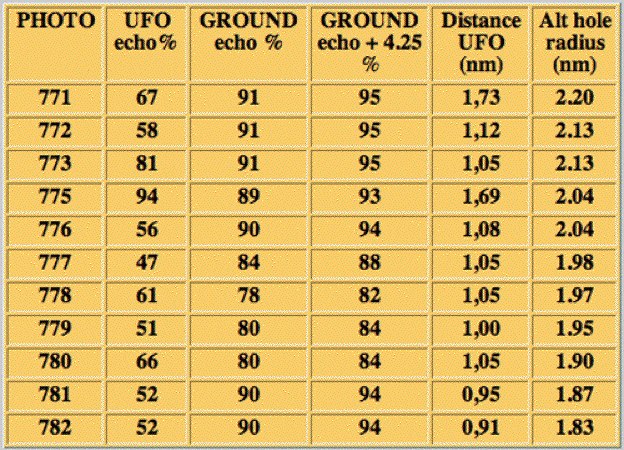Table 8. Comparison of photometric measurements with the signal from a virtually perfect reflector on the ground. The third column (GROUND echo + 4.25%) shows the corrected value for a 100% reflecting ground.

Now let us consider the following equation derived from equation (57), where φ = 90° and D = 2.20 nm, corresponding to the corrected ground echo of photo

95 = -19.96 - 13.52 log {sin(α)} + 27.04 log (2.20 )}         (58)

This calculation generates a correct angle Alpha that is close to zero. We have determined from equation (58) that:

• -13.52 log {sin(α)} = 105.7

Therefore, equation (58) becomes:

95 = -19.96 + 105.7 + 27.04 log (2.20)}         (59)

This is 95 = 95, and therefore true. Let us consider the following equation derived from equation (57), where φ = 90° and D = 1.73 nm corresponding to the UFO echo of photo 771

67 = -19.96 - 13.52 log {sin(α)} + 27.04 log (1.73)}         (60)

Finally, let us subtract (60) from (59):

95 - 67 = 95 + 19.96 + 13.52 log {sin(α)} - 27.04 log (1.73)}         (61)

Therefore,

67 + 19.96 + 27.04 log (1.73)} = -13.52 log {sin(α)}         (62)

This equation should generate the value of the angle a for the UFO in photo 771, although we find a ≈ 0 degrees. This is normal since Ed% for the UFO is always smaller than the corrected value of Ed% for the ground, except in photo 775 where equation (62) becomes:

94 + 19.96 + 27.04 log (1.69)} = -13.52 log {sin(α)}         (63)

This equation should generate the value of the angle a for the UFO in photo 775, but again we find, a ≈ 0 degrees for photo 775.

Finally, all a angles calculated this way are equal to zero, because there is a need for a very high attenuation of the returned signal to explain why the UFO echo is much smaller than the ground echo, particularly when the UFO distance to the B-52 is always smaller than the ground distance. This means that our previous hypothesis concerning the radar albedo of the UFO is false, because there is considerable absorption of the radar microwaves beam in the direction of the UFO. We can calculate this absorption by correcting the value of Ed%, so that the UFO would be at the same distance as the ground-altitude hole edge. If we look at equation (54), we see that:

Ed% = Constant + 4 γ log (D)         (64)

If D becomes nD, the value of ED% is reduced by 4 γ log ( n ), with:

n = D (ground) / D (UFO)         (65)

In Table 9, the seventh column provides the value of n. Now the distance correction for the UFO is as follows:

ΔEd% = -27.04 log (n)         (66)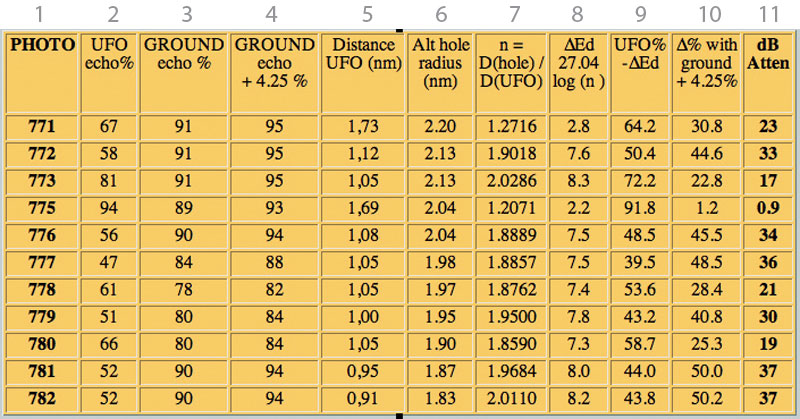Table 9. Calculation of the attenuation of the UFO returned radar echo by the surrounding ion cloud.

The eighth column provides the value of ΔEd%. By subtracting this (negative) value from the value of the UFO echo% in column 2, we receive the corrected Ed% for the UFO in column 9. This is the value of the photo darkening we would see if the UFO were situated at the same distance as the altitude hole edge. By subtracting column 9 from column 4, we get column 10, which is the calculated UFO echo attenuation expressed in photo darkening. We find a relatively large attenuation because it corresponds to a variation of the photo emulsion darkening in the order of 50% of the ground echo in the photos.

However, we have to remember that the photographic emulsion generates a darkening that is proportional to the logarithm of the screen echo brightness. Determining the value of the attenuation expressed in decibels (dB Atten) requires a multiplication of column 10 by 20 / 4γ to get column 11. The column 11 values are the attenuation in decibels of the radar signal if the UFO albedo is assumed to be 100% in the center of an absorbing cloud. In fact, the size of the UFO is so large that if it were made of metal, it should have returned a much brighter echo than the ground echo close to the altitude hole edge, since the UFO is always closer to the B-52 than to the ground. But this is not what the photos show, except photo 775 where the UFO echo is approximately as bright as the ground echo. In all other photos, the attenuation of the echo compared to the echo in photo 775 is in the order of 29 decibels on average, which is a relatively large attenuation.

Because we had not yet calibrated the whole system (radar + photos), we made the assumption that the radar camera was linear, much like a modern CCD camera. However, we see now that this is not the case. Instead, the B-52 radar camera was logarithmic. Therefore, the UFO echo attenuation can no longer be explained by the UFO movement, which would change the value of the α angle, and give corresponding radar antenna attenuations. This does not necessarily signify that the UFO did not move up and down, but rather that the resulting attenuation from a change of this angle is quite small compared to the 29 decibels we have calculated. This is shown in Figure 49, where the antenna attenuations (a few decibels) are indicated versus angle α.The only explanation we find for this attenuation of 29 dB is a thick cloud of ionized air surrounding the UFO. The presence of this cloud is coherent with the visual observation of the luminosity of the UFO, and with the interruption of the VHF transmissions on two occasions when the B-52 was close or closer than about one nautical mile. The oversized radar echo is also coherent in comparison to the various witness descriptions of the UFO size.

In summary, the photogrammetric and photometric study we have undertaken on photographic copies of the original B-52 radarscope photos has increased the accuracy of the reconstruction of the radar observation. It has allowed us to determine the most probable B-52 descent trajectory, and it has given the most probable value (50°) for the radar antenna tilt-up angle. An accurate mathematical model of the whole system of the radar photos has been derived that provides a confirmation of the presence of a large ionized cloud of gas surrounding the UFO.

## Conclusion

It is certainly interesting to consider that this apparently non-aerodynamic aerial device has no comparison with all currently known technological developments. Here again, we are dealing in one or more devices in which the dynamics and energy characteristics are quite simply phenomenal, and have the theoretical potential to allow for an interstellar voyage.

Effectively, the maximum UFO acceleration deduced from the B-52 radarscope photos (400 g's) allows for a relativistic speed (more than 90% of the speed of light) in a relatively short time (less than a day). This is one of the two indispensable conditions for traveling between stars, since it allows sufficient relativistic time “compression” for the crew to arrive at the destination during their life span. The second indispensable condition is the ability to produce a tremendous amount of energy onboard the spacecraft, something the Minot UFO has demonstrated in its powerful light emissions, and phenomenal maneuvers.

In this case, we also have a panoply of interesting physical effects, including the extreme luminosity of the air, loss of the B-52 VHF transmissions on two discrete occasions, and a powerful radar echo. We will probably never know all the facts, but what we have been able to reconstruct is perfectly clear from my point of view, and reinforces what I have written on the site www.universons.com/.

The conclusions of Blue Book in 1968 appear perplexingly ridiculous, however, it really doesn’t matter since the truth is inherent. And this truth implicates knowledge of considerable potential for the future of humanity. We need to join efforts to understand the physics revealed, since it is by the union of our knowledge that we will advance our understanding.

Dr. Claude POHER
Toulouse, France
August 6, 2005

Appendix 1 ››